# One half 2

One half pizza will be divide among 3 pupils. Each pupil receive 1/6. Is it true or false?

Result

x=

### Step-by-step explanation: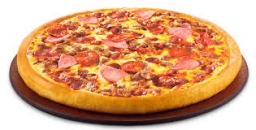Did you find an error or inaccuracy? Feel free to write us. Thank you!Tips to related online calculators
Need help to calculate sum, simplify or multiply fractions? Try our fraction calculator.

## Related math problems and questions:

• Pizza 5You have 2/4 of a pizza, and you want to share it equally between 2 people. How much pizza does each person get?
• Barbara 2Barbara get 6 pizzas to divide equally among 4 people. How much of a pizza can each person have?
• Pie division5/8 of a pie divide 6 pieces. Each friend got 1/6.  What fraction  of the whole pie does each person receive?
• Pizza 4Marcus ate half pizza on monday night. He than ate one third of the remaining pizza on Tuesday. Which of the following expressions show how much pizza marcus ate in total?
• Pizza fractionsAnn ate a third of a pizza and then another quater. Total part of pizza eaten by Ann and how much pizza is left?
• A pizza 2A pizza is cut into slices that are each 1/6 of the whole. John is going to eat 1/2 of the whole pizza. How many slices will John eat?
• Pizza cover5/8 of the pizza is covered in mushrooms, 1/4 of the pizza is covered with pineapples, and the rest of the pizza is covered with only cheese. What fraction of the pizza is covered with only cheese?
• Divide 11Divide the product of 4 and 5/8 by 1 1/2. Write your answer as a mixed number.
• 80 students80 students were asked what type of test they preferred. 50 students said they liked multiple choice and 42 liked true or false. If 36 liked both multiple choice and true or false types, how many students preferred multiple choice only?
• JerryJerry has 3/4 of a pizza. He needs to share it with 6 friends. What fraction of the pizza will each friend get? Only write the fraction
• Divide 13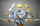Divide. Simplify your answer and write as an improper fraction or whole number. 14÷8/3
• IssacIssac eats 1/6 of the pizza. Maya then eats 3/5 of the remaining pizza. What fraction of the original pizza is left?
• Slices of pizzaMaria ate 1/4 of a pizza. If there were 20 slices of pizza, how many slices did Maria eat?
• Pizza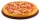Three siblings ordered one pizza. Miška ate a quarter of the whole pizza. Lenka ate a third of the rest and Patrik ate half of what Lenka had left. They had the rest packed up. How much of the pizza did they pack? Write the result as a fraction.
• School year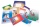At the beginning of the school year, 396 notebooks and 252 textbooks are ready to be distributed in the classroom. All pupils receive the same number of notebooks and the same amount of textbooks. How many pupils are there in the class if you know that th
• Rings groups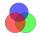27 pupils attend some group; dance group attends 14 pupils, 21 pupils sporty group and dramatic group 16 pupils. Dance and sporting attend 9 pupils, dance and drama 6 pupil, sporty and dramatic 11 pupils. How many pupils attend all three groups?
• Julian 2Julian and two of his friends are going to share 1/4 of a pizza. How much will each person get?